or# RD Sharma Class 12 and Chapter Adjoints And Inverse Of Matrix Maths Solutions

## Maths Class 12 and Chapter Adjoints And Inverse Of Matrix RD Sharma SolutionsFilter
Filters :
CLASSES
•
•
•
•
•
•
•
CHAPTERS
•
• 24 More

As much as it's true that no one can change these facts about NCERT books, the utmost need of an expert's help guides having detailed explanations and good to start with the notes is also a reality that most of the students can't deny. You must be thinking which the most liked help is the guide that students adore the most, then the answer is RD Sharma help books.

Recommended by most of the experienced teachers and educational experts, RD Sharma 12th class maths book is not less than a blessing for students who want to step ahead of fixed NCERT formulas and want to learn all the tricky concepts of adverse and inverse of a matrix.

The book indeed has a vast number of questions and examples that enhances the attitude of the student and make him able to solve complex problems in a minute of time. Not only this, but every single chapter of 12th maths is explained thoroughly with lots of solved and unsolved questions to practice from.

Not to mention but students will also be able to find accurate solutions of miscellaneous exercises given at the end of each chapter in NCERT.

Because according to experienced teachers and council of the CBSE board, most of the questions come directly from the exercises given in the NCERT books. And that's why it is important to have precise answers with step by step solutions.

Remember, every step count in the CBSE board exams and even a slightest of mistake can cost you a deduction of marks. So, choose wisely and get your RD Sharma book today.

## INTRODUCTION:

Adjoint Matrix of a square matrix can be defined as the transpose of the matrix formed by the co-factors of the elements of determinant |A|.

To calculate the adjoint of the matrix we must follow the procedure:

a) Calculate the Minor for each element.

b) Form Cofactor matrix from the minors calculated.

c) Form Adjoint from cofactor matrix.

EXAMPLE 2.6.11   Let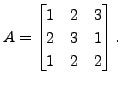Then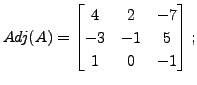### INVERSE OF MATRIX

The inverse of a square matrix A, known as a reciprocal of the matrix, is a matrix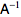such that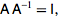(1)

Where I is the identity matrix. Courant and Hilbert use the symbol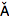to denote the inverse matrix.

A square matrix A has an inverse if the determinant |A|is not equal to zero. The invertible matrix theorem is the main result in linear algebra which associates the existence of a matrix inverse with a number of other equivalent properties. A matrix having an inverse is called non-singular, or invertible of the matrix.

In the Wolfram Language, The matrix inverse of a square matrix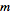may be called the function Inverse[m].

For a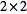matrix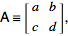the matrix inverse is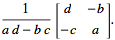For a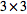matrix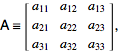the matrix inverse is

### The Relation between Adjoint and Inverse of a Matrix

To find the inverse of a matrix A, i.e A-1 we shall first define the adjoint of a matrix. Let A be an n x n matrix. The (i,j) cofactor of A is defined to be

Aij = (-1)ij det(Mij),

where Mij is the (i,j)th minor matrix obtained from A after removing the ith row and jth column. Let’s consider the n x n matrix A = (Aij) and define the n x n matrix Adj(A) = AT. The matrix Adj(A) is called the adjoint of matrix A. When A is invertible, then its inverse can be obtained by the formula given below.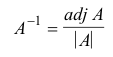The inverse is defined only for non-singular square matrices. This relationship is between a matrix and its inverse:

AA-1 = A-1A = I, where I is the identity matrix.

## Theorems on Adjoint and Inverse of a Matrix

### Theorem 1

If A be any given square matrix of order n, then A adj(A) = adj(A) A = |A|I, where I is the identity matrix of order n.

Proof: Let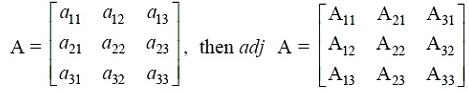Since the sum of the product of elements of a row (or a column) with corresponding cofactors is equal to |A| and otherwise zero, we have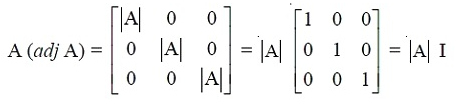From the above, we can now show that adj(A) A = |A| I

### Theorem 2

If A and B are non-singular matrices of the same order, then AB and BA are also non-singular matrices of the same order.

### Theorem 3

The determinant of the product matrices is equal to the product of their respective determinants, that is, |AB| = |A||B|, where A and B are square matrices of the same order.

We know that,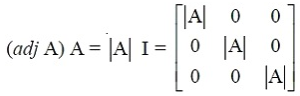using determinants of matrices on both sides, now we have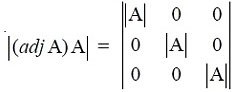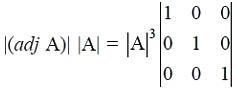In general, if A is a square matrix of order n, then |adj(A)| = |A|n-1.

### Theorem 4

A square matrix A is invertible if and only if A is a non-singular matrix.

Proof: Let A be an invertible matrix of order n and I be the identity matrix of the same order. Then there exists a square matrix B of order n such that AB = BA = I. Now, AB = I.

So |A| |B| = |I| = 1 (since |I| = 1 and |AB| = |A| |B|). This gives |A| to be a non-zero value. Hence A is a non-singular matrix. Conversely, let A be a non-singular matrix, then |A| is non-zero. Now A adj(A) = adj(A) A = |A| I (Theorem 1). Or,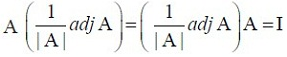or AB = BA = I, where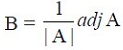Thus, A is invertible and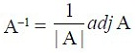## IMPORTANT NOTES:

Here, we list without proof some of the most important rules of matrix algebra - theorems that rule the way that matrices are added, multiplied, and otherwise manipulated.

### Matrix Addition and Matrix Multiplication

• A + B = B + A (Commutative law of addition)

• A + B + C = A + (B + C) = (A + B) + C (Associative law of addition)

• ABC = A (BC) = (AB)C (Associative law of multiplication)

• A (B + C) = AB + AC (Distributive law of matrix algebra)

• x (A + B) = xA + xB

### Transposition Rules

• (A’)' = A

• (A + B)' = A' + B'

• (AB)' = B'A'

• (ABC)' = C'B'A'

### Inverse Rules

• AI = IA = A

• AA-1 = A-1A = I

• (A-1)-1 = A

• (AB)-1 = B-1A-1

• (ABC)-1 = C-1B-1A-1

## IMPORTANCE OF RD SHARMA CLASS 12TH Math

1. The 12th standard Mathematics RD Sharma help students to brush up their concepts right from the base and to understand the theme of the topics.

2. The students who continuously referred RD Sharma help guides to unleash the route to success because it gives basic concepts rather than solving the formula-based questions directly.

3. We all know that maths is not literature type subject that we can learn Instead, it is a subject that requires timely learning of formulas and practicing the questions as much as a student caliber allows.

4. RD Sharma 12th class maths books have multiple practice questions & exercises to solve to better understand the main concept of question and how to solve it.

5. Abstracted and collected by education sector experts, these books are structured as per the current exam patterns. The new edition of the book contains all the recent changes and are double checked by experienced teachers.

6. As the book consists of both, solved and unsolved questions, the students can find the solutions of such practice exercises online as well. In short, RD Sharma books are compiled nicely and aided with online solutions.